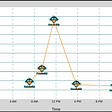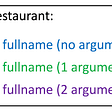# Regular Expressions : RegEx

`.       - Any Character Except New Line\d      - Digit (0-9)\D      - Not a Digit (0-9)\w      - Word Character (a-z, A-Z, 0-9, _)\W      - Not a Word Character\s      - Whitespace (space, tab, newline)\S      - Not Whitespace (space, tab, newline)\b      - Word Boundary\B      - Not a Word Boundary^       - Beginning of a String\$       - End of a String[]      - Matches Characters in brackets[^ ]    - Matches Characters NOT in brackets|       - Either Or( )     - GroupQuantifiers:*       - 0 or More+       - 1 or More?       - 0 or One{3}     - Exact Number{3,4}   - Range of Numbers (Minimum, Maximum)`
`import retext_to_search = '''abcdefghijklmnopqurtuvwxyzABCDEFGHIJKLMNOPQRSTUVWXYZ1234567890123abcHello HelloHelloMetaCharacters (Need to be escaped):. ^ \$ * + ? { } [ ] \ | ( )gmail.com321-555-4321123.555.1234abhi-arya@gmail.comMr. JohnsonMr SmithMs DavisMrs. RobinsonMr. T'''`
`pattern = re.compile(r'cba')matches = pattern.finditer(text_to_search)for mat in matches:    print(mat)Output: Will search for the literals 'cba' in the text.`

# Searching special characters

## Different special characters:

`pattern = re.compile(r'.')matches = pattern.finditer(text_to_search)for mat in matches:    print(mat)Output: Any Character Except New Line`
`pattern = re.compile(r'\.')matches = pattern.finditer(text_to_search)for mat in matches:    print(mat)`
`pattern = re.compile(r'\D')matches = pattern.finditer(text_to_search)for mat in matches:    print(mat)pattern = re.compile(r'\d\w')matches = pattern.finditer(text_to_search)for mat in matches:    print(mat)pattern = re.compile(r'\d\s')matches = pattern.finditer(text_to_search)for mat in matches:    print(mat)`

# Word boundary

`# Hello HelloHellopattern = re.compile(r'Hello') #searching for 'Hello'matches = pattern.finditer(text_to_search)for mat in matches:    print(mat)`
`<re.Match object; span=(74, 79), match='Hello'><re.Match object; span=(80, 85), match='Hello'><re.Match object; span=(85, 90), match='Hello'>`
`pattern = re.compile(r'Hello\b') #Word Boundarymatches = pattern.finditer(text_to_search)for mat in matches:    print(mat)`
`<re.Match object; span=(74, 79), match=’Hello’> <re.Match object; span=(85, 90), match=’Hello’>Note: Here we searched for the pattern were 'Hello' was followed by word boundary(space/new line etc.)`
`pattern = re.compile(r'\bHello\b')matches = pattern.finditer(text_to_search)for mat in matches:    print(mat)`
`<re.Match object; span=(74, 79), match='Hello'>`
`Other Worth trying examples:pattern = re.compile(r'\BHello\b') # \B - Not word boundarymatches = pattern.finditer(text_to_search)for mat in matches:    print(mat)pattern = re.compile(r'\b\d')matches = pattern.finditer(text_to_search)for mat in matches:    print(mat)pattern = re.compile(r'^\s') #Whitespace (space, tab, newline) at beginning of stringmatches = pattern.finditer(text_to_search)for mat in matches:    print(mat)`

# Character sets

`pattern = re.compile(r'\w')matches = pattern.finditer(text_to_search)for mat in matches:    print(mat)`
`<re.Match object; span=(55, 57), match='12'><re.Match object; span=(57, 59), match='34'><re.Match object; span=(66, 68), match='12'><re.Match object; span=(68, 70), match='3a'><re.Match object; span=(169, 171), match='32'><re.Match object; span=(178, 180), match='32'><re.Match object; span=(182, 184), match='12'><re.Match object; span=(190, 192), match='12'><re.Match object; span=(192, 194), match='34'>`
`pattern = re.compile(r'[a-z][a-z]')matches = pattern.finditer(text_to_search)for mat in matches:    print(mat)pattern = re.compile(r'[a-zA-Z0-9][a-zA-z-]')matches = pattern.finditer(text_to_search)for mat in matches:    print(mat)pattern = re.compile(r'[a-zA-Z][^a-zA-z]')matches = pattern.finditer(text_to_search)for mat in matches:    print(mat)`

# Character groups

`pattern = re.compile(r'(abc|com|texas)\b')matches = pattern.finditer(text_to_search)for mat in matches:    print(mat)pattern = re.compile(r'([A-Z]|llo)[a-zA-z]')matches = pattern.finditer(text_to_search)for mat in matches:    print(mat)`

# Quantifiers

`pattern = re.compile(r'Mr\.?\s[A-Z]')matches = pattern.finditer(text_to_search)for mat in matches:    print(mat)pattern = re.compile(r'Mr\.?\s[A-Z][a-z]*')matches = pattern.finditer(text_to_search)for mat in matches:    print(mat)pattern = re.compile(r'M(s|rs)\.?\s[A-Z][a-z]*')matches = pattern.finditer(text_to_search)for mat in matches:    print(mat)pattern = re.compile(r'\d{3}[.-]\d{3}[.-]\d{4}')matches = pattern.finditer(text_to_search)for mat in matches:    print(mat)pattern = re.compile(r'[a-zA-Z0-9_]+\.[a-z]{3}')matches = pattern.finditer(text_to_search)for mat in matches:    print(mat)pattern = re.compile(r'[a-zA-Z0-9_.+-]+@[a-zA-Z0-9-]+\.[a-zA-Z0-9-.]+')matches = pattern.finditer(text_to_search)for mat in matches:    print(mat)`

# Accessing information in the Match object

`pattern = re.compile(r'[a-zA-Z0-9_.+-]+@[a-zA-Z0-9-]+\.[a-zA-Z0-9-.]{2,4}')matches = pattern.finditer(text_to_search)for mat in matches:    print(mat.span(0))    print(mat.group(0))    print(text_to_search[mat.span(0):mat.span(0)])urls = r'''https://www.google.comhttp://yahoo.comhttps://www.whitehouse.govhttps://craigslist.org'''pattern = re.compile(r'https?://(www\.)?\w+\.\w+')matches = pattern.finditer(urls)for mat in matches:    print(mat)pattern = re.compile(r'https?://(www\.)?(\w+)(\.\w+)')matches = pattern.finditer(urls)for mat in matches:    print(mat.group(2)+mat.group(3))pattern = re.compile(r'https?://(www\.)?(\w+)(\.\w+)')matches = pattern.finditer(urls)for mat in matches:    print(mat.group(0))    print(urls[mat.span(2):mat.span(2)]+urls[mat.span(3):mat.span(3)])`

--

--

--

## More from Abhinandan Arya

Senior Applied Scientist | Satellite, Space and Earth Observation (EO) Data

Love podcasts or audiobooks? Learn on the go with our new app.

## Snowflake Deep Dive## Understanding MockK Kotlin## Learn FlutterJS and start your journey## Scaling a Kubernetes Cluster: One of the Best Practices for Using KubeKey## Graphing Time Series Data through Unity## Twitch Integrations | Part 2## All You Need to Know About Databases## Abhinandan Arya

Senior Applied Scientist | Satellite, Space and Earth Observation (EO) Data

## IBM Brazil's Marathon Behind the Code 2021